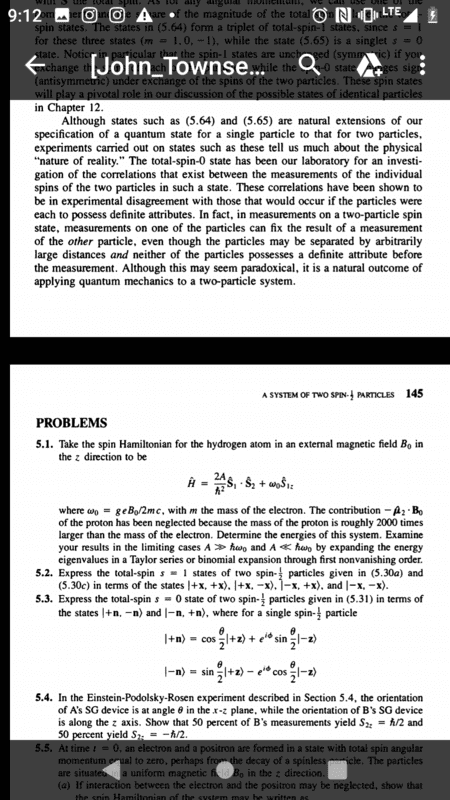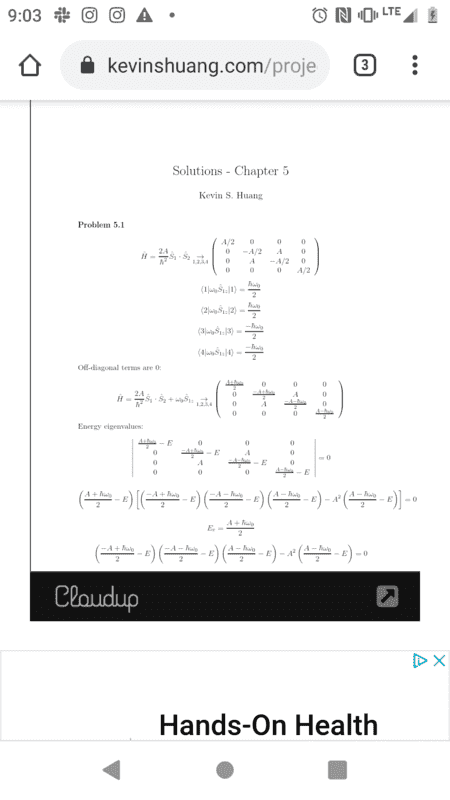# Spin Hamiltonian of a hydrogen atom in a magnetic field

Homework Statement:
5.1 of the first picture is the homework statement and the second is the solution. So my question is in the solution, how did they compute <1|w_0S_z|1>?
Relevant Equations:
The book uses Dirac notation for matrix computation but this homework is not for a class and is strictly for recreation so feel free to solve it using the mathematical methods you feel comfortable with.qa

Thus, consider a state ##|\Psi \rangle = |\alpha_1 \rangle |\beta_2 \rangle## in which the electron is in some spin state ##|\alpha_1 \rangle## while the proton is in spin state ##|\beta_2 \rangle##. Then $$\langle \Psi|\hat S_{1z}|\Psi \rangle = \langle \beta_2|\langle \alpha_1|\hat S_{1z }\otimes \mathbb {I}_2|\alpha_1 \rangle |\beta_2 \rangle = \langle \alpha_1|\hat S_{1z }|\alpha_1\rangle \langle \beta_2|\mathbb {I}_2|\beta_2\rangle = \langle \alpha_1|\hat S_{1z }|\alpha_1\rangle \langle \beta_2|\beta_2\rangle$$When you get used to this, you will mentally go directly from the far left to the far right of this series of steps without having to write the intermediate steps.